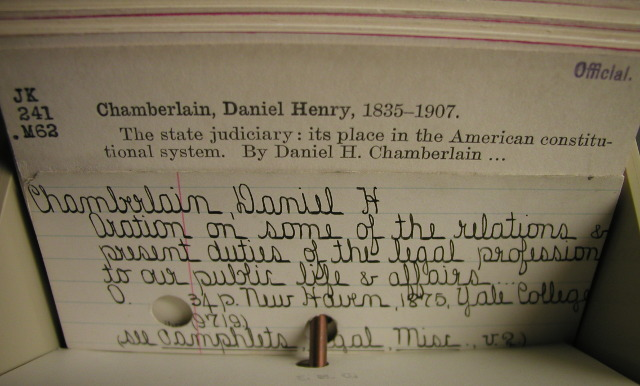# Create, initialize and compare structs

yourbasic.org/golang## Struct types

A struct is a typed collection of fields, useful for grouping data into records.

``````type Student struct {
Name string
Age  int
}

var a Student    // a == Student{"", 0}
a.Name = "Alice" // a == Student{"Alice", 0}
``````
• To define a new struct type, you list the names and types of each field.
• The default zero value of a struct has all its fields zeroed.
• You can access individual fields with dot notation.

## 2 ways to create and initialize a new struct

The `new` keyword can be used to create a new struct. It returns a pointer to the newly created struct.

``````var pa *Student   // pa == nil
pa = new(Student) // pa == &Student{"", 0}
pa.Name = "Alice" // pa == &Student{"Alice", 0}
``````

You can also create and initialize a struct with a struct literal.

``````b := Student{ // b == Student{"Bob", 0}
Name: "Bob",
}

pb := &Student{ // pb == &Student{"Bob", 8}
Name: "Bob",
Age:  8,
}

c := Student{"Cecilia", 5} // c == Student{"Cecilia", 5}
d := Student{}             // d == Student{"", 0}
``````
• An element list that contains keys does not need to have an element for each struct field. Omitted fields get the zero value for that field.
• An element list that does not contain any keys must list an element for each struct field in the order in which the fields are declared.
• A literal may omit the element list; such a literal evaluates to the zero value for its type.

For further details, see The Go Language Specification: Composite literals.

## Compare structs

You can compare struct values with the comparison operators `==` and `!=`. Two values are equal if their corresponding fields are equal.

``````d1 := Student{"David", 1}
d2 := Student{"David", 2}
fmt.Println(d1 == d2) // false
``````

### Go step by stepCore Go concepts: interfaces, structs, slices, maps, for loops, switch statements, packages.